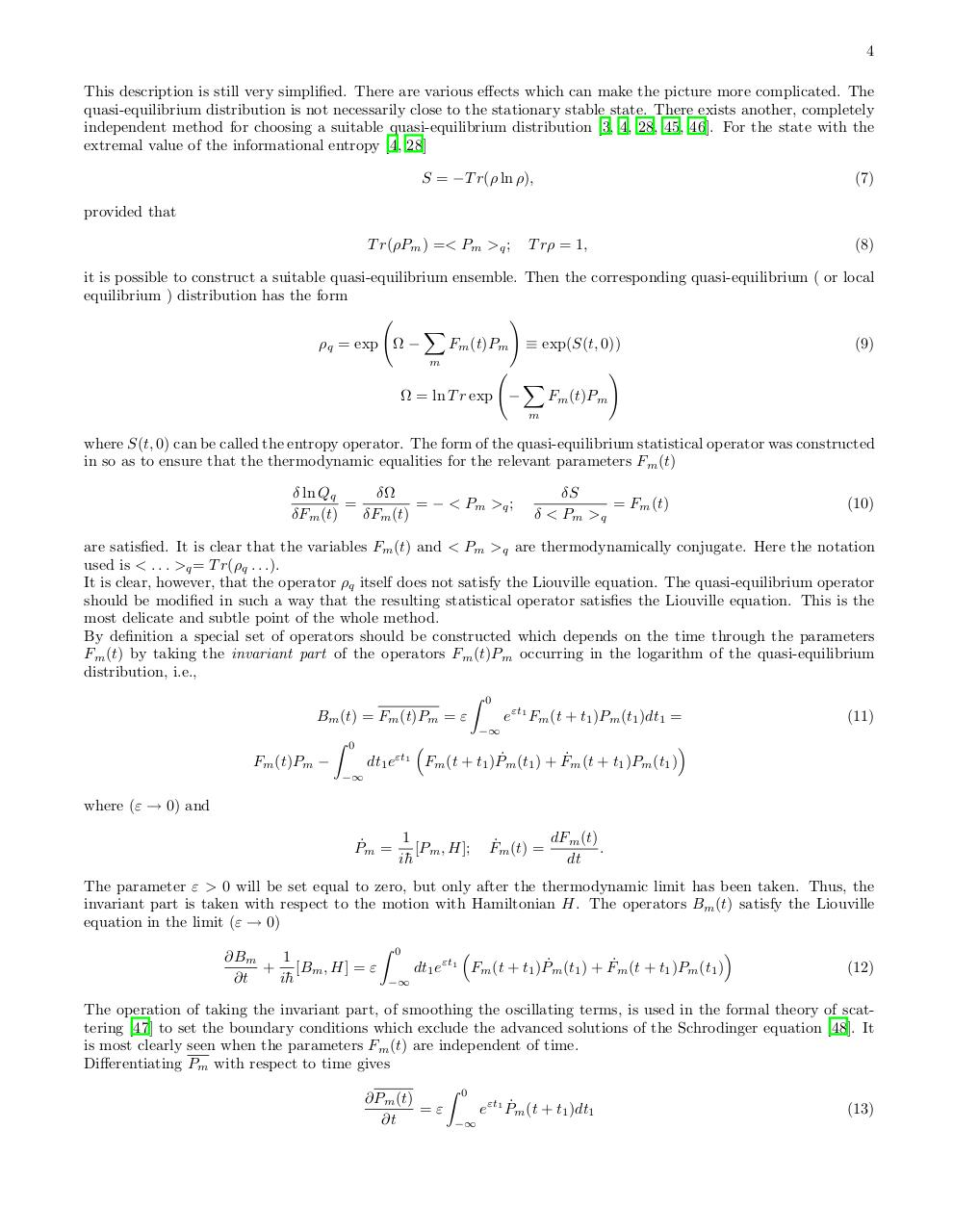PDF Archive

Easily share your PDF documents with your contacts, on the Web and Social Networks.

Nonequilibrium Statistical Operator IJMPB05.pdfPage 1 2 3 45621

Text preview

4
This description is still very simplified. There are various effects which can make the picture more complicated. The
quasi-equilibrium distribution is not necessarily close to the stationary stable state. There exists another, completely
independent method for choosing a suitable quasi-equilibrium distribution [3, 4, 28, 45, 46]. For the state with the
extremal value of the informational entropy [4, 28]
S = −T r(ρ ln ρ),

(7)

provided that
T r(ρPm ) =&lt; Pm &gt;q ;

T rρ = 1,

(8)

it is possible to construct a suitable quasi-equilibrium ensemble. Then the corresponding quasi-equilibrium ( or local
equilibrium ) distribution has the form
!
X
(9)
ρq = exp Ω −
Fm (t)Pm ≡ exp(S(t, 0))
m

Ω = ln T r exp −

X

Fm (t)Pm

m

!

where S(t, 0) can be called the entropy operator. The form of the quasi-equilibrium statistical operator was constructed
in so as to ensure that the thermodynamic equalities for the relevant parameters Fm (t)
δ ln Qq
δΩ
=
= − &lt; Pm &gt;q ;
δFm (t)
δFm (t)

δS
= Fm (t)
δ &lt; Pm &gt;q

(10)

are satisfied. It is clear that the variables Fm (t) and &lt; Pm &gt;q are thermodynamically conjugate. Here the notation
used is &lt; . . . &gt;q = T r(ρq . . .).
It is clear, however, that the operator ρq itself does not satisfy the Liouville equation. The quasi-equilibrium operator
should be modified in such a way that the resulting statistical operator satisfies the Liouville equation. This is the
most delicate and subtle point of the whole method.
By definition a special set of operators should be constructed which depends on the time through the parameters
Fm (t) by taking the invariant part of the operators Fm (t)Pm occurring in the logarithm of the quasi-equilibrium
distribution, i.e.,
Z 0
Bm (t) = Fm (t)Pm = ε
(11)
eεt1 Fm (t + t1 )Pm (t1 )dt1 =
−∞

Fm (t)Pm −

Z

0

−∞

dt1 eεt1 Fm (t + t1 )P˙m (t1 ) + F˙m (t + t1 )Pm (t1 )

where (ε → 0) and
1
P˙m = [Pm , H];
i¯h

dFm (t)
F˙m (t) =
.
dt

The parameter ε &gt; 0 will be set equal to zero, but only after the thermodynamic limit has been taken. Thus, the
invariant part is taken with respect to the motion with Hamiltonian H. The operators Bm (t) satisfy the Liouville
equation in the limit (ε → 0)
1
∂Bm
+ [Bm , H] = ε
∂t

h

Z

0

−∞

dt1 eεt1 Fm (t + t1 )P˙m (t1 ) + F˙m (t + t1 )Pm (t1 )

(12)

The operation of taking the invariant part, of smoothing the oscillating terms, is used in the formal theory of scattering  to set the boundary conditions which exclude the advanced solutions of the Schrodinger equation . It
is most clearly seen when the parameters Fm (t) are independent of time.
Differentiating Pm with respect to time gives
∂Pm (t)

∂t

Z

0

−∞

eεt1 P˙m (t + t1 )dt1

(13)